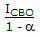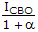# Electronics and Communication Engineering - Electronic Devices and Circuits

6.

The conductivity of materials found in nature ranges from 109 ohm-1m-1 to nearly 1018 ohm-1 m-1 from this it can be concluded that the conductivity of silicon in ohm-1 cm-1 will be nearly

 A. 0.5 x 10-15 B. 0.5 x 10-21 C. 0.5 x 10-12 D. 0.5 x 10-3

Answer: Option D

Explanation:

No answer description available for this question. Let us discuss.

7.

At any point on the v-i characteristics of a semiconductor diode, the slope of v-i characteristics is called

 A. resistance of diode B. conductance of diode C. incremental resistance of diode D. incremental conductance of diode

Answer: Option D

Explanation:

No answer description available for this question. Let us discuss.

8.

The collector to emitter cutoff current (ICE0) of a transmitter is related to collector to base cut off current (ICB0) as

 A. ICE0 = ICB0 B. ICE0 = a ICB0 C. ICE0 =D. ICE0 =Answer: Option C

Explanation:

No answer description available for this question. Let us discuss.

9.

Which of the following statement about the photo electric emission is incorrect?

 A. The maximum velocity of emission varies with the frequency of incident light B. The maximum velocity of emission varies with the intensity of light C. The amount of photoelectric emission is directly proportional to the intensity of light D. The quantum yield depends on the frequency and not the intensity of incident light

Answer: Option B

Explanation:

No answer description available for this question. Let us discuss.

10.

In a semiconductor avalanche breakdown occurs when

 A. reverse bias exceeds the limiting value B. forward bias exceeds the limiting value C. forward current exceeds the limiting value D. potential barrier is reduced to zero

Answer: Option A

Explanation:

No answer description available for this question. Let us discuss.

#### Current Affairs 2021

Interview Questions and Answers